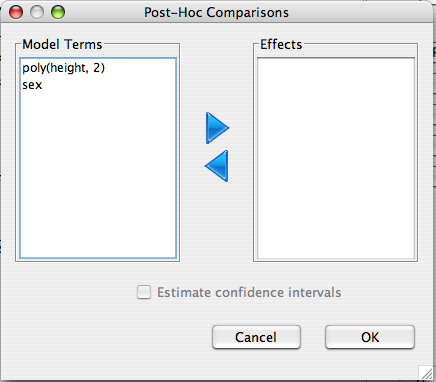Much like the effects plots, the marginal means are the estimated model based means for the outcome variable across the levels of a term given that the other variables are fixed at a typical level (i.e. thier mean).

## Dialog